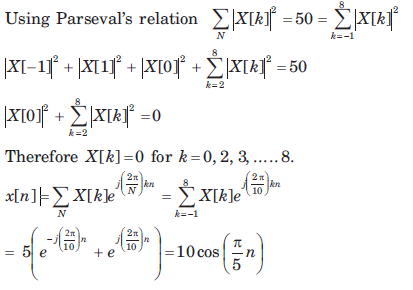Courses

# The Discrete -Time Fourier Series - MCQ Test

## 15 Questions MCQ Test Mock Tests Electronics & Communication Engineering GATE 2020 | The Discrete -Time Fourier Series - MCQ Test

Description
This mock test of The Discrete -Time Fourier Series - MCQ Test for Railways helps you for every Railways entrance exam. This contains 15 Multiple Choice Questions for Railways The Discrete -Time Fourier Series - MCQ Test (mcq) to study with solutions a complete question bank. The solved questions answers in this The Discrete -Time Fourier Series - MCQ Test quiz give you a good mix of easy questions and tough questions. Railways students definitely take this The Discrete -Time Fourier Series - MCQ Test exercise for a better result in the exam. You can find other The Discrete -Time Fourier Series - MCQ Test extra questions, long questions & short questions for Railways on EduRev as well by searching above.
QUESTION: 1

### Consider a periodic signal x[n] with period N and FS coefficients X [k]. Determine the FS coefficients Y [k] of the signal y[n] given in question. Que: y[n] = x[ n - no]

Solution: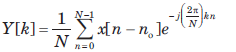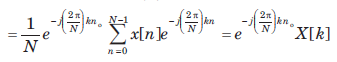QUESTION: 2

### Consider a periodic signal x[n] with period N and FS coefficients X [k]. Determine the FS coefficients Y [k] of the signal y[n] given in question. y[n] = x[n] - x[n-2 ]

Solution: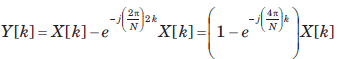QUESTION: 3

### Consider a periodic signal x[n] with period N and FS coefficients X [k]. Determine the FS coefficients Y [k] of the signal y[n] given in question. Que: y[n] =  x[n] + x[n + N/2 ] , (assume that N is even)

Solution:

Note that y[n] =  x[n] + x[n + N/2 ] has a period of N/2 and N has been assumed to be even,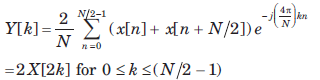QUESTION: 4

Consider a periodic signal x[n] with period N and FS coefficients X [k]. Determine the FS coefficients Y [k] of the signal y[n] given in question.

Que: y[n] = x[n] - x[n + N/2 ] , (assume that N is even)

Solution: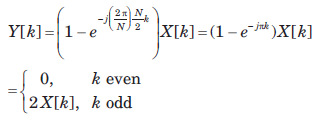QUESTION: 5

Consider a periodic signal x[n] with period N and FS coefficients X [k]. Determine the FS coefficients Y [k] of the signal y[n] given in question.

Que: y[n] = x*[-n]

Solution:

y[n] = x*[-n]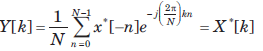QUESTION: 6

Consider a periodic signal x[n] with period N and FS coefficients X [k]. Determine the FS coefficients Y [k] of the signal y[n] given in question.

y[n] = (-1)nx[n], (assume that N is even)

Solution:

With N even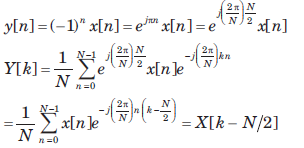QUESTION: 7

Consider a discrete-time periodic signal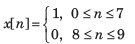with period  N = 10, Also y[n] = x[n] - x[n-1]

Que: The fundamental period of y[n] is

Solution:

y[n] is shown is fig.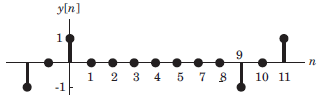It has fundamental period of 10.

QUESTION: 8

Consider a discrete-time periodic signalwith period  N = 10, Also y[n] = x[n] - x[n-1]

Que: The FS coefficients of y[n] are

Solution: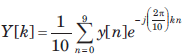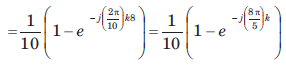QUESTION: 9

Consider a discrete-time periodic signalwith period  N = 10, Also y[n] = x[n] - x[n-1]

The FS coefficients of x[n] are

Solution: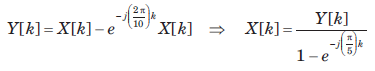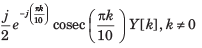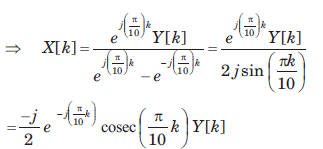QUESTION: 10

Consider a discrete-time signal with Fourier representation.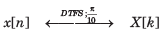In question the FS coefficient Y [k] is given. Determine the corresponding signal y[n] and choose correct option.

Que: Y[k] = X[k-5] + X[k+5]

Solution: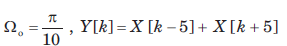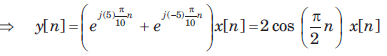QUESTION: 11

onsider a discrete-time signal with Fourier representation.In question the FS coefficient Y [k] is given. Determine the corresponding signal y[n] and choose correct option.

Que: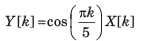Solution: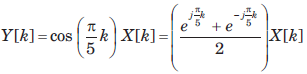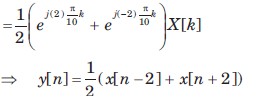QUESTION: 12

Consider a discrete-time signal with Fourier representation.In question the FS coefficient Y [k] is given. Determine the corresponding signal y[n] and choose correct option.

Que: Y[k] = X[k]*X[k]

Solution: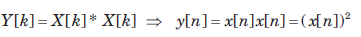QUESTION: 13

Consider a discrete-time signal with Fourier representation.In question the FS coefficient Y [k] is given. Determine the corresponding signal y[n] and choose correct option.

Que: Y[k] = Re{X[k]}

Solution: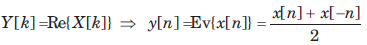QUESTION: 14

A real and odd periodic signal x[n] has fundamental period N = 7 and FS coefficients X [k]. Given that X  = j, X  = 2j, X  = 3j. The values of X ,X [-1], X [-2], and X [ -3] will be

Solution:

Since the FS coefficient repeat every N. Thus

X = X, X = X, X = X
The signal real and odd, the FS coefficient X[k] will be purely imaginary and odd. Therefore X = 0 X[-1] = -X, X[-2] = -X, X[-3]  = -X[-3]
Therefore (D) is correct option.

QUESTION: 15

Consider a signal x[n] with following facts

1. x[n] is a real and even signal

2. The period of x[n] is N = 10

3. X  = 5​ ​ ​ ​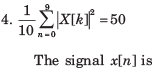Solution:

Since N = 10, X = X = 5
Since x[n] is real and even X[k] is also real and even.
Therefore X = X[-1] = 5.